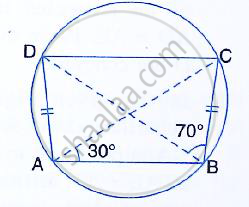Share

In the Figure, Given Below, Ad = Bc, ∠Bac = 30° and ∠Cbd = 70°. Find: (I) ∠Bcd (Ii) ∠Bca (Iii) ∠Abc (Iv) ∠Adb - ICSE Class 10 - Mathematics

ConceptArc and Chord Properties - Angles in the Same Segment of a Circle Are Equal (Without Proof)

Question

In the figure, given below, AD = BC, ∠BAC = 30° and ∠CBD = 70°.
Find:   (i) ∠BCD (ii) ∠BCA (iii) ∠ABC (iv) ∠ADBSolution

In the figure, ABCD is a cyclic quadrilateral
AC and BD are its diagonals

∠BAC = 30°  and ∠CBD = 70°

Now we have to find the measure of
∠BCD, ∠BCA, ∠ABC and ∠ADB
We have ∠CAD = ∠CBD = 70°  [Angles in the same segment] Similarly, ∠BAD = ∠BDC = 30°
= 30°  + 70°
= 100°

(i) Now ∠BCD + ∠BAD =180°  [Opposite angles of cyclic quadrilateral]
⇒ ∠BCD + ∠BAD = 180°
⇒ ∠BCD + 100°  = 180°
⇒ ∠BCD = 180°  -100°
⇒ ∠BCD = 80°

(ii) Since AD = BC, ABCD is an isosceles trapezium and AB ∥ DC

∠BAC = ∠DCA    [Alternate angles]
⇒ ∠DCA = 30°

∴ ∠ABD = ∠DAC = 30°  [Angles in the same segment]
∴ ∠BCA = ∠BCD - ∠DAC
= 80°  - 30°
= 50°

(iii)  ∠ABC = ∠ABD + ∠CBD
= 30°  + 70°

= 100°
(iv) ∠ADB = ∠BCA = 50°   [Angles in the same segment]

Is there an error in this question or solution?
Solution In the Figure, Given Below, Ad = Bc, ∠Bac = 30° and ∠Cbd = 70°. Find: (I) ∠Bcd (Ii) ∠Bca (Iii) ∠Abc (Iv) ∠Adb Concept: Arc and Chord Properties - Angles in the Same Segment of a Circle Are Equal (Without Proof).
S